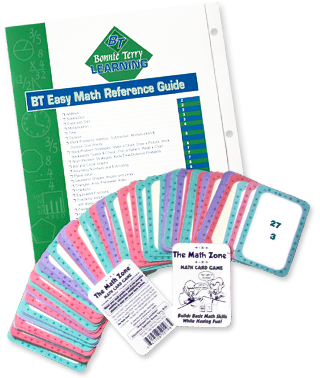# The Math Pack Arithmetic Game, a Math Reference Guide, and Paper Organizers for Math Homework Help

The Bonnie Terry Math Pack consists of a game (The Math Zone™), a guide (The BT Easy Math Reference Guide), and Super Spacers (20 paper organizers) that address the 3 roadblocks to math success Plus a FREE Bonus.

Unfortunately, this product is out of stock.

• Add, subtract, multiply, and divide.
• Calculate fractions, decimals, and percents.
• Calculate area, perimeter, and ratio.
• Recognize and do calculations with geometric shapes, lines, and angle.
• Find the clue words for word problems.
• Understand math vocabulary.

## The Math Zone

### A Memory and Math Concepts Game

A calculation practice game where children add, subtract, multiply, divide, halve the answer, or double their answers. Players learn how numbers grow and shrink with different mathematical calculations.

## The BT Easy Math Reference Guide

### The Ultimate Guide for Math

This math homework help guide gives children of all ages, from elementary school through college, the code, secrets, and tools they need to solve all elementary math calculations right up to fractions, decimals, and percents.

## Super Spacers

### 2 Packs of 20 Paper Organizers

Gives children of all ages perfect spacing for math problems. Now they can have neat and organized papers!

## Bonus

A FREE Tip Sheet on getting the best use of the BT Easy Math Reference Guide (Valued at \$5.95).

Imagine how confident and proud your kids will feel about themselves when they are able to do their math homework on their own and get it right! Imagine your child actually enjoying math, even playing a math game. They can even learn to solve word problems with ease, while enjoying doing them, because they will be interested in the story of the problem. That is why my daughter likes to do math problems – she likes the stories! Your child will be able to understand math vocabulary, so solving problems will be like child’s play. And their assignment will even be done neatly, where answers to problems don’t run into each other. This can happen… this can be your child too!

#### Guarantee

As with all Bonnie Terry Learning Products, The Math Pack comes with a 30-Day Money Back Guarantee.